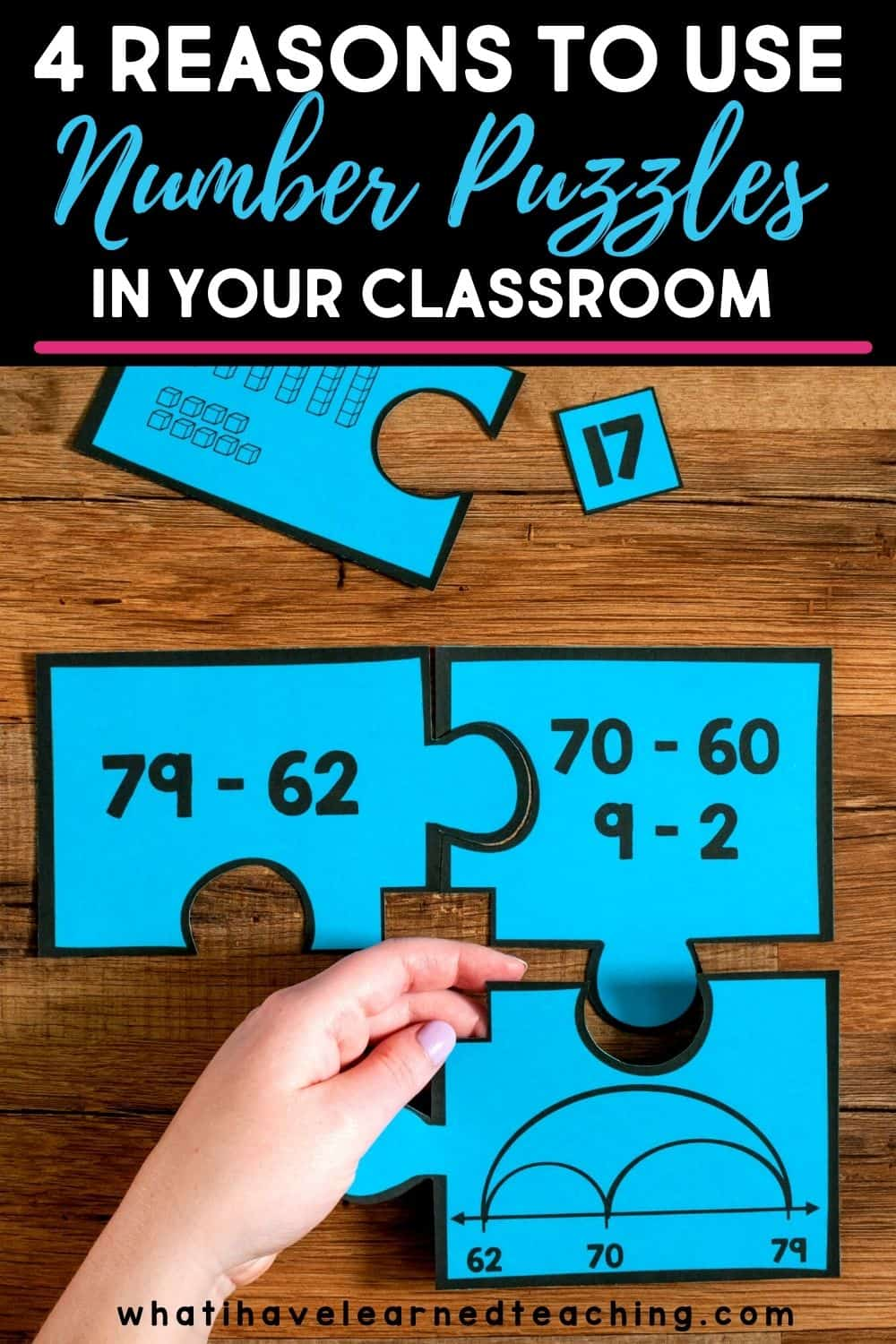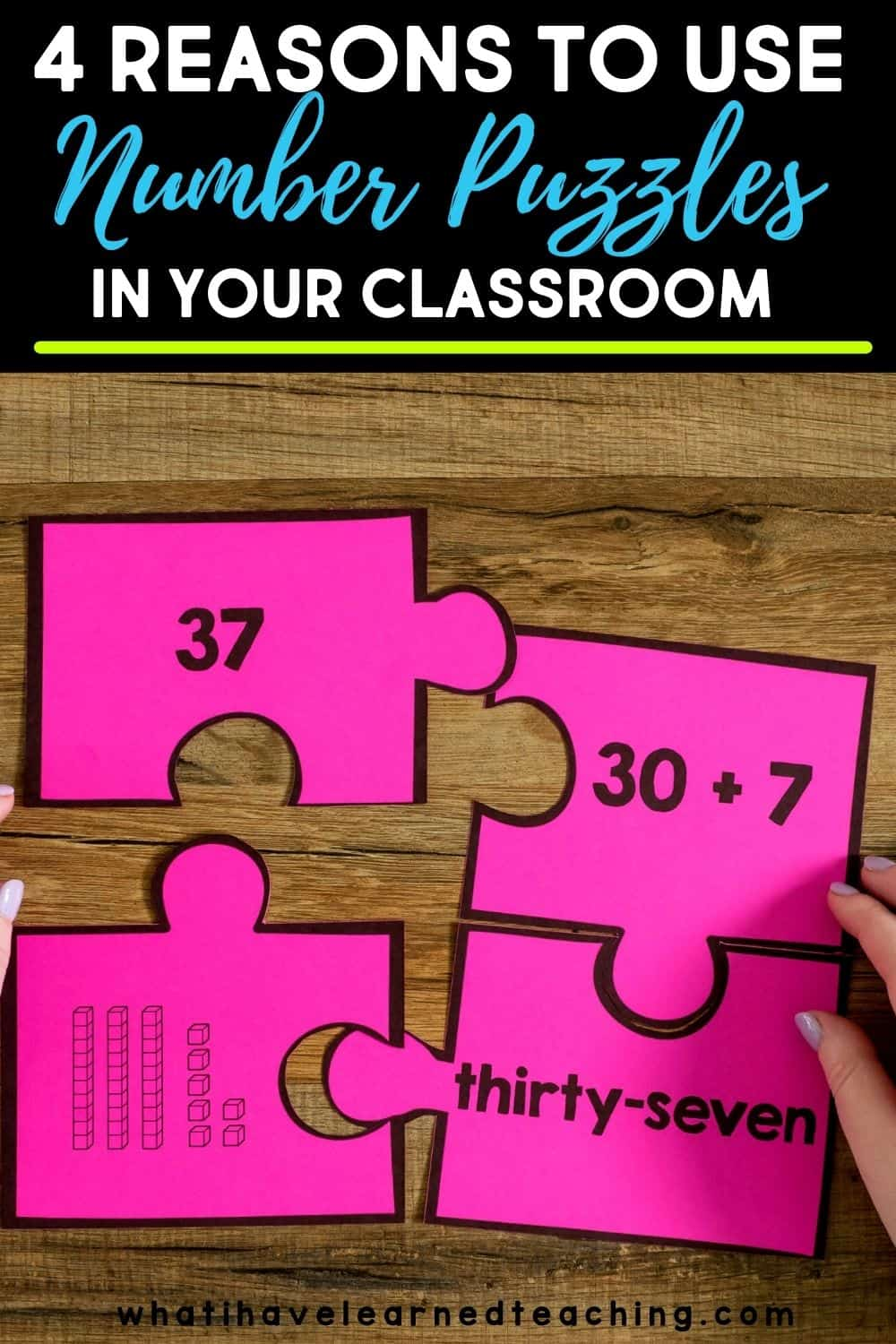Tuesday June 6, 2023
Latest:
198 total views

Math is not just reading equations and coming up with the answers. Remember, math is all about the process. Number puzzles help your students think about different ways to work with numbers to form equations and generate answers. There are so many reasons to use number puzzles in your classroom!

## What are number puzzles?

To begin, number puzzles are cut-out puzzle pieces that fit together to form different expressions of the same number. For example, my two-digit number puzzles model four different ways to solve a two-digit addition problem. The five puzzle pieces include the addition expression, the numbers broken apart by place value, base-10 blocks, and a number line. Finally, the answer is a square that goes in the middle of all four pieces.

### The number puzzle bundle includes the following puzzles for second grade:

• Using 10 to Add Number Puzzles
• Subtraction Number Puzzles (Two-Digit)
• Decompose Two-Digit Number Puzzles
• Money Number Puzzles
• Place Value Number Puzzles (Two- & Three-Digit)
• Subtraction Number Puzzles (Three-Digit, same as third grade)
• Odd / Even Number Puzzles
• Time Puzzles
• Fractions Number Puzzles
• Multiplication Number Puzzles

• Multiplication Interpretation
• Division Interpretation
• Multiplication & Division Word Problems
• Determine Unknown Numbers for Multiplication and Division Equations
• Apply Properties of Operations – Multiplication & Division
• Relationship Between Multiplication & Division
• Two-Step Word Problems
• Rounding to the Nearest 10 & 100
• Subtraction (Three-Digit, same for second grade)
• Multiply by Multiples of 10
• Fraction Number Lines, Visual Models & Compare Fractions Number Puzzles
• Time to the One Minute & Interval Word Problems Number Puzzles
• Area & Perimeter

## Why should you use number puzzles in your classroom?

Now that you know what number puzzles are, why should you use them in your classroom instruction? There are so many benefits to number puzzles for your primary learners!

### Hands-On Cooperative Learning

First, try using number puzzles in math centers! Number puzzles are literally hands-on learning! For example, I love using number puzzles for differentiated small groups. Give students puzzles based on their skill level. Putting together the puzzles to see how the equations develop in different ways is even better when your students can have conversations and work together cooperatively.

When your students are putting together the puzzles, you’ll hear their conversations about how the expressions relate to each other. Of course, this metacognition process is so important in math!

### The Answer is Given and Needs to be Matched

Again, number puzzles are all about the process and expressions that lead to an answer. Each puzzle already has an answer given. The students’ job is to find the expressions that all lead to that answer. Whether the strategies used are the equation expression, base-10 blocks, expanded form, word form, or place value, your students will be able to recognize different ways to find the given answer.

### Develop Flexible Thinking in Math

Of course, actually thinking about math and problem-solving is so valuable in the classroom. Number puzzles allow your students to examine problems and the ways to find answers in different ways. By really reflecting on the process of math and thinking about the steps, your students will be able to practice and develop flexible thinking skills!

### Use as Independent Practice

Although I love using number puzzles for cooperative work, they are also fantastic for independent practice and review. For example, if your students are at home with remote learning, send the link to the puzzles for additional practice. When your students are back in the classroom, you’ll love using number puzzles for early finishers or extra review for specific skills!

Overall, using number puzzles for your primary learners will help your young learners develop metacognitive skills, work together to problem-solve, and get independent practice. Try these number puzzles in your classroom!### News

Some days, you simply have to put the books aside and head outside. These nature crafts are the perfect…